Web of Science, ISC, CAS, Google Scholar

Document Type : Original Article

Authors

Department of Chemical Engineering, Quchan Branch, Islamic Azad University, Quchan, Iran

Abstract

In this study, a thermodynamic behavior of the quaternary system of (Poly propylene fumarate/ (Graphene oxide/ Pluronic F-68))/2-pyrrolidone (2P)/water was evaluated. The binodal and spinodal curves plotted using by Flory–Huggins theory and compared with experimental data. The results showed that at low value of binary parameter, a high interaction is existed between two components in ternary system. The ternary system at positive ternary interaction parameter (g123) indicates a small miscible region and small changes in water content of the polymer-rich phase. At the negative values of g123, there is a high miscibility in ternary system. In quaternary system, when g1234 is positive, the binodal curve shifts to the (polymer/additive)/ solvent axis. The membrane formation depends on the thermodynamic behavior of casting solution. By increasing the additive in casting solution up to 4wt.%, the de-mixing process was accelerated. Hence, a membrane was formed with high porosity. When additive content was increased to more than 4 wt%, dynamic viscosity of casting solution was responsible to the phase separation rate decreasing.

Graphical Abstract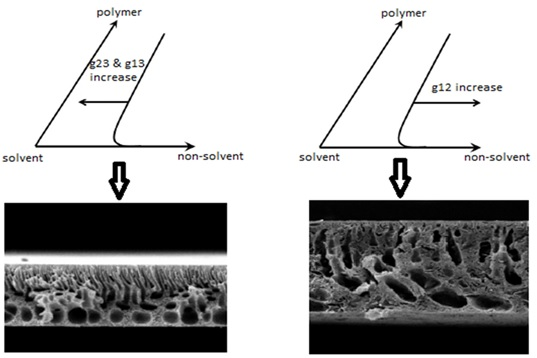Keywords

Main Subjects

###### ##### Full Text

Introduction

Phase inversion is one of methods used in polymeric membrane construction. Polymer based membranes are of interest for separation technology [1-3].

Thermodynamic evaluation of the casting solution should be done before preparing the membrane [4, 5]. This assessment is possible by examining the phase diagrams and gives us an overview of the membrane structure .

Flory Huggins theory is used as a widely used theory in the thermodynamic study of concentrated solutions and polymer mixtures . The phase diagram of the membrane solution for three- or four-component is mentioned as binodal curve in the ternary or quaternary diagram. The Flory-Huggins involves ternary and quaternary interaction parameters of each component in membrane solution.

To calculate the Flory-Huggins interaction parameters, the solubility parameters of the components are used . The thermodynamic behavior of casting solution can be obtained by binodal curve position and miscibility gap area evaluation in ternary or quaternary phase diagram. The position of bonodal curve related to the several parameters such as interaction parameters of three or four components and a composition of the casting solution [9, 10]. The influence of those parameters on the membrane structure have been explained in some literature [11, 12].

In phase diagram, when the binodal composition is reached, non-solvent molecules take the place of solvent molecules and phase separation of the membrane solution begins to occur. With more solvent and non-solvent exchange, the membrane solidification begins and the process of membrane formation is completed . In the blend membranes, polymer concentration, the blending ratio and solvent and non-solvent play a main role in thermodynamic stability of the prepared membrane . However, the different additives using in the casting solution affects the structure of the membrane. Hence, the presence of the suitable additive in the casting solution has an effect on the kinetic and thermodynamic properties of the casting solution and is also effective on the membrane formation mechanism [15-18].

This study aimed to predict the thermodynamic behavior of (Poly propylene fumarate/ (Graphene oxide/ Pluronic F-68))/2-pyrrolidone (2P)/ water quaternary system. Therefore, the ternary and quaternary interaction parameters predicted and analyzed. Likewise, the effect of interaction parameters on membrane morphology was investigated.

Materials and Methods

Poly propylene fumarate (Mw = 3600 g/mol) was supplied by Sigma Aldrich/Germany. Solvent of 2-pyrrolidone (2P) (>99%) was purchased from Merck/Germany. Pluronic F-68 (Mw = 1000 gr/mol, PEO weight content: %80, poly (propyl oxide) weight content:20%) was purchased from BASF (Germany). Graphene oxide (GO) was purchased from ACS Material (USA).

Binodal and spinodal curves by experimental method

The ternary phase diagram of the PPF/2-pyrrolidone/water combination systems was determined by cloud point measurement. Therefore, at first an appropriate amount of PPF was placed in glass-ware reactor under stirring by heating at 60 ᵒC for about 1.5 h. Thereafter, the water was slowly added to the PPF solutions until turbidity disappears. The ternary phase diagram was obtained from the turbid points. These points with the different compositions of components make visual measurement of bimodal curve at ternary diagram. Furthermore, by further water increasing, the solution was separated into the distinct solid and liquid phases. These points made the experimental spinodal curve. To calculate the quaternary system, the polymeric solution was prepared after dispersing GO/PF-68 in 2P by sonication.

Binodal and spinodal curves by theoretical method

Figure 1 displays the ternary phase diagram for the polymer/ solvent/non-solvent ternary system. At point A, the casting solution only consists of solvent, polymer, and additives. In fact, there is a single-phase region from point A to point B. At point B, the solvent precipitates out and non-solvent molecules take its place and the single phase turns into two phases. At point C, the membrane begins to solidify, and at point D, the process is complete. The binodal curve defines the boundary between the single-phase and two-phase regions. Likewise, by the spinodal curve, the unstable region restricted and the single phase turns into two phases. From the collision of spinodal and binodal curves, a critical point is created. The thermodynamic instability of the system determines by the distance between the bimodal curve and solvent-polymer axis. For the theoretical evaluation of binodal and spinodal curves, the Flory-Huggins theory is extensively used.

Gibbs free energy of mixing () for ternary and quaternary polymer solution are given by following equations :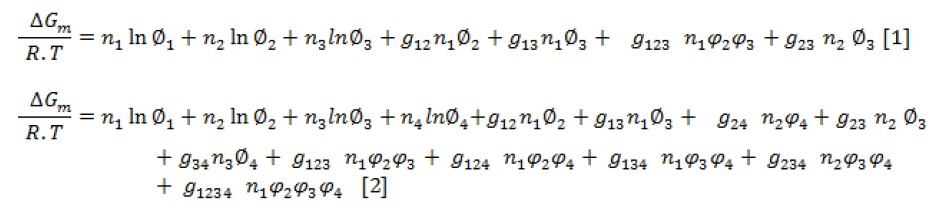Where, ni denotes the molar number,  is the volume fraction,  is the binary interaction parameter, is the ternary interaction parameter, and is the quaternary interaction parameter. The subscripts 1, 2, 3, and 4 represent non-solvent, solvent, polymer, and additive, respectively.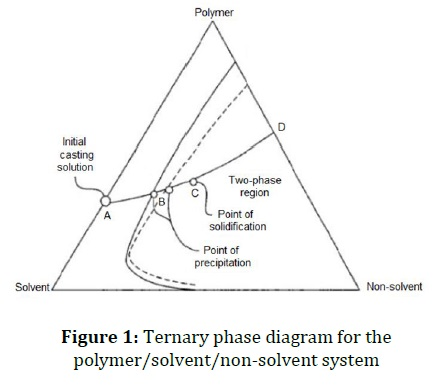Ternary system evaluation

To define the binodal curve, the chemical potential of the polymer-rich phase and that of the polymer-lean phase achieve equilibrium. This equilibrium in a ternary system consisting of non-solvent (1), solvent (2), and polymer (3) are as follows:Where, A and B indices indicate polymer-lean and polymer-rich phases.

According to the definition of chemical potential , the following three formulas are used to calculate the chemical potentials of components .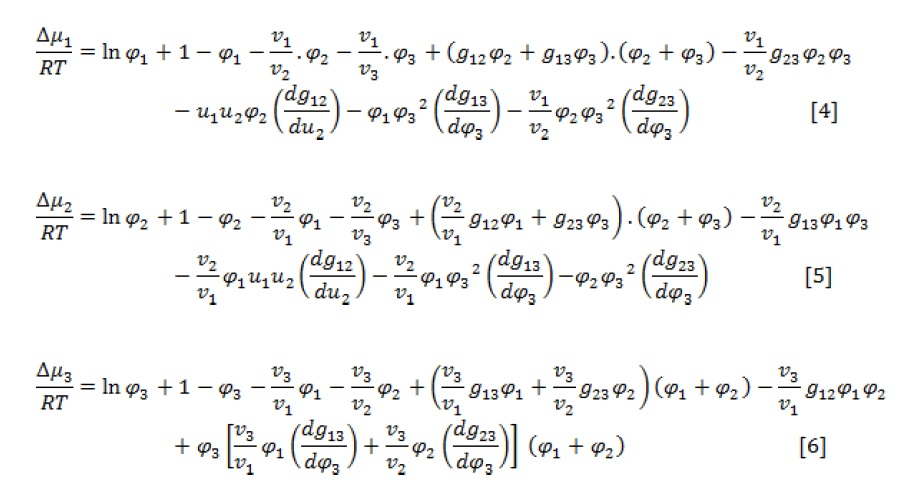Where, vi is the molar volume of component i and is the difference of component i chemical potential in the mixture and pure state. Also,According to the material conservation relations, there is Equation 7 for each component :Equations (3-7) are five coupled nonlinear equations with six unknown variables:,, ,,, and . To solve five non-linear equations, one composition of the component is considered as an independent value.

Since the amount of polymer in the polymer-lean phase was very small, is assumed negligible.

For spinodal curve evaluation, the following equation is used :The expressions of G22, G23, and G33, as free energies, are as follow:The spinodal curve is obtained by simultaneously solving equations 7 and (8-12) and given a parameter as an independent variable.

Quaternary system evaluation

The following equations are used to evaluate a quaternary polymer/additive/solvent/non-solvent system :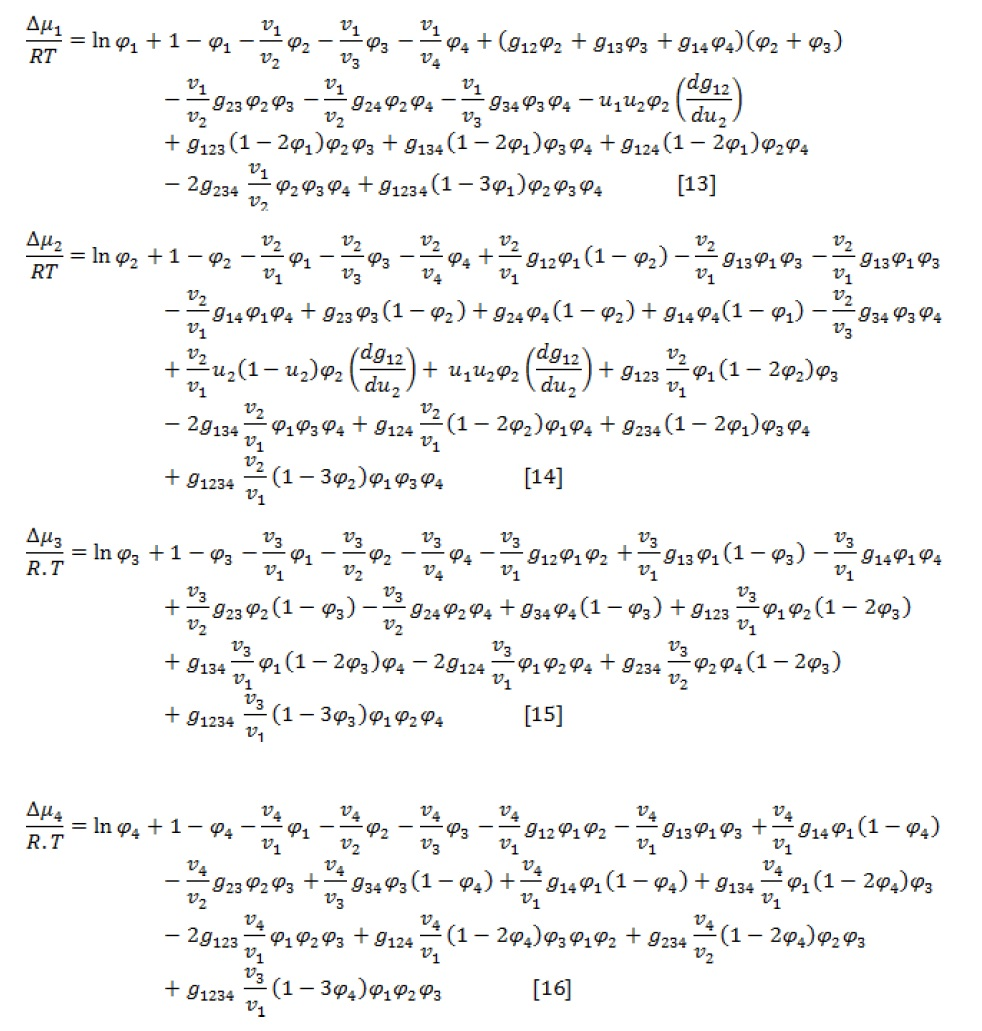Calculation of non-solvent-solvent interaction parameter

Based on many researches, the interaction parameters for two-component solvent-non-solvent systems are given by the following equation:Where,  is the concentration-dependent interaction parameter in the non-solvent-solvent system, , is the volume fraction of solvent, and , , and  are three parameters varied for various systems.

The small interaction parameter of  represents the extreme polar interactions between the solvent and non-solvent in ternary system.

Interaction parameter calculation of (non-solvent)-polymer

To estimate the interaction parameter of (non-solvent)-polymer, several experimental methods such as absorption, osmotic pressure, equilibrium swelling, and light scattering have been used, among which the equilibrium swelling method is a simple, common, and effective method. The Flori-Renner theory is used to describe the expression of membrane swelling. The Flori-Renner equation in expressing membrane swelling behavior is expressed by the following general relation:Where, ,  ,, and  are the volume fraction of the polymer in the swollen system, crosslink density (volume/mol), solvent or non-solvent molar volume, and the Flori-Renner parameter (which represents the energy of the solvent or non-solvent interaction with the polymer), respectively.

When the solvent has a weak interaction with the polymer so that the weight increase of the polymer after swelling is not high (less than 30% of its initial weight), the free elastic enthalpy in the free energy change is negligible and is not considered. Therefore, Equation 12 changes as follows: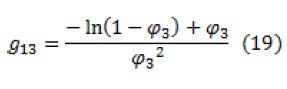Where,  is the volume fraction of the polymer and is calculated by the following equation: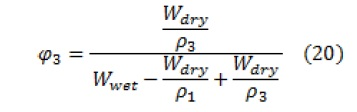In the above equation, indices 1, 2, and 3 are related to the solvent, non-solvent, and polymer, respectively.

Interaction parameter calculation of solvent-polymer

There are various methods to calculate the solvent-polymer interaction parameter (. These methods are including osmotic pressure, light scattering, liquid-gas equilibria, etc. among which laboratory liquid-gas equilibrium is usually preferred. However, in low solvent partial pressures, this method is not used. The solvent-polymer interaction parameter can be estimated by following equation: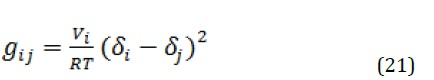Where,  is the molar volume of the solvent and  and are the solubility parameters of components i and j, respectively.

According to the ternary solubility parameter theory proposed by Hansen, the solubility parameter for the polymer can be estimated with the molar gravitational constant (F) of different groups in repeating units, as described by the following equations:Where, is the molar volume of all groups in the structural unit,  represents the energy required to overcome the scattering forces,  represents the energy required to overcome the polar interactions between adjacent molecules, and  represents the energy required to break the hydrogen bonds between adjacent molecules.

Membrane preparation and characterization

Phase inversion method was used to prepare asymmetric PPF membrane . A stainless steel casting knife with a gap distance of 250 μm was used to cast the casting solution on the mirror plate at ambient temperature (evaporation time: 20 second), and then the glass plate was subsequently immersed immediately in deionized water. After the formation of the membrane, it is removed from the non-solvent bath and washed with distilled water several times to remove the residual solvent. The SEM images of the prepared membranes were prepared by the KYKY EM3200.

Results and Discussion

Binary interaction parameters effect on ternary system

The Binary interaction parameters of Flory-Huggins theory play an important role in the solubility of each component in the casting solution and determine the position of the binodal curve. Therefore, the low value of this parameter indicates a high interaction between two components in ternary system. If there is a high interaction between the solvent and the polymer, g23 has a low value. Likewise, the lower g14 shows a high interaction between non-solvent and additive. Since pluronic F-68/GO has a hydrophilic nature, it causes more penetration of water flow into the nascent membrane and thermodynamic instability. Therefore, the binodal curve is more shift the polymer/additive/solvent axis, which indicates the greater instability of the system as a result of the presence of the additive. In contrast, the high value of this parameter indicates a low interaction between two components in ternary system. At higher value of g13, the binodal curve tends towards the (polymer/additive) - solvent axis in ternary diagram. The summery result of this section is displayed in Figure 2.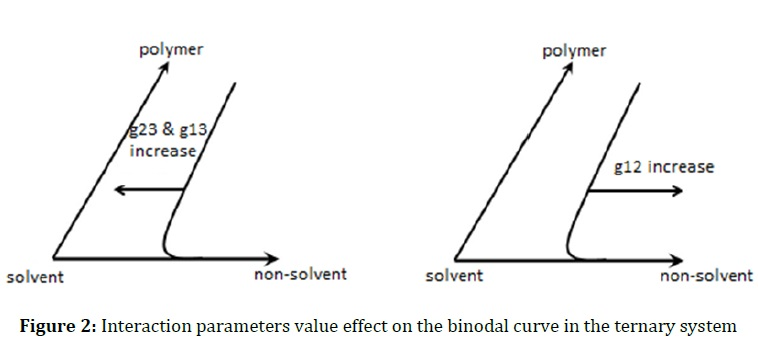The value obtained for the g24 =1.02 indicates that the additive is well disperse in the solvent. In fact, the hydrophobic chain in the Pluronic F-68 additive (i.e. poly (propylene oxide)) improves the dispersion of graphene in the non-polar solutions. Since g13, g23, and g14 have a great effect on the liquid-liquid and solid-liquid demixing behavior of the ternary system, the other binary interaction parameters (g12 and g23) are ignored.

Ternary interaction parameters effect on ternary system

Figure 3 displays the calculated binodal curve for the PPF/2P/Water system at various g123 from - 0.5 to +0.5 (T = 25 °C). As can be seen, when g123 is positive, the system shows a small miscible region and small changes in water volume fraction of the polymer-rich phase. Therefore, the small amounts of non-solvent are needed for phase separation. Conversely, due to a negative value of g123 indicating a high miscibility in ternary system, the binodal curve will shift toward the polymer/non-solvent axis in the ternary diagram as a result of Gibbs free energy reduction so that phase separation is minimized.

Figure 4 depicts the ternary phase diagrams of PPF/2P/Water systems at g123 of 0.56 which are obtained from the cloud-point measurements, binodal, spinodal, and critical point calculations. The experimental results show a good agreement with the theoretical binodal results. Thus, the value of 0.56 was considered for g123 parameter in the ternary system.Quaternary interaction parameters effect on quaternary system

The effect of the quaternary interaction parameters on the binodal curve in the quaternary (PPF/additive)/2P/Water system is depicted in Figure 5.

When g1234 is positive, the addition of additive into the casting solution disturb the thermodynamic equilibrium of system and leads to instability. Therefore, the binodal curve shifts to the polymer/additive/ solvent axis. It might be explained by that the hydrophilic nature of the pluronic F-68/GO increases water as a non-solvent inflow and cases lower thermodynamic stability. Likewise, the binodal curves showed at different ternary parameters in quaternary system (Figure 5b-d). It is clear that there are negligible interactions of pluronic F-68/GO with solvent and polymer (g234) at various ternary parameters in the system (Figure 5b). Both g124 and g134, at positive value are close to (polymer/additive)/solvent axis and conversely at negative value are shift to (polymer/additive)/non-solvent axis. In fact, the pres­ence of special relation (interaction and affinity) between pluronic F-68/GO and water as the non-solvent provides a high permeation velocity of non-solvent into nascent film during the phase inversion and disturbs the thermodynamic stability. Moreover, the solvent diffusion from the membrane to the coagulant can be further affected by the pluronic F-68/GO.

As a result of the presence of additive, molecular interactions between polymer and solvent are reduced so that the diffusion of the solvent from the poly­mer matrix in to coagulant becomes easier. All of this causes the binodal curve to shift to the left of quaternary system.Morphology of membranes

The cross-sectional SEM image of the modified and unmodified membranes is illustrated in Figures 6 and 7.

Since, PPF is amorphous; it is cross-sectional SEM image with a finger like structure. This structure is obtained in the composition near to the critical point. Likewise, the finger like structure with a dense top-layer and porous sub-layer is exhibited in modified membranes (Figure 7).

A great diffusion velocity of water into nascent membrane is occurred during the phase inver­sion in presence of GO/Pluronic F-68 additive, so macrovoids grow throughout the membrane.

The loading of Pluronic F-68 causes homogenous dispersion of GO in the polymer matrix and prevents the aggregation of GO so that the result is a more regular formation of macrovoids in the membrane structure.

The presence of Pluronic F-68 affects the micelle structure of the casting solution so that the density of the micelles influences the membrane structure. Based on the results, by increasing GO/Pluronic F-68 up to 4 wt.%, the size of the micelles increased, resulting in the formation of membranes with a macroporous support layer, a dense skin layer, and a porous sub-layer.

At higher additive concentration (>4wt.%), a dense sponge-like structure of sub-layer was obtained. Furthermore, the finger-like structure of the support layer was transformed into larger pores and extended to the bottom of the membrane.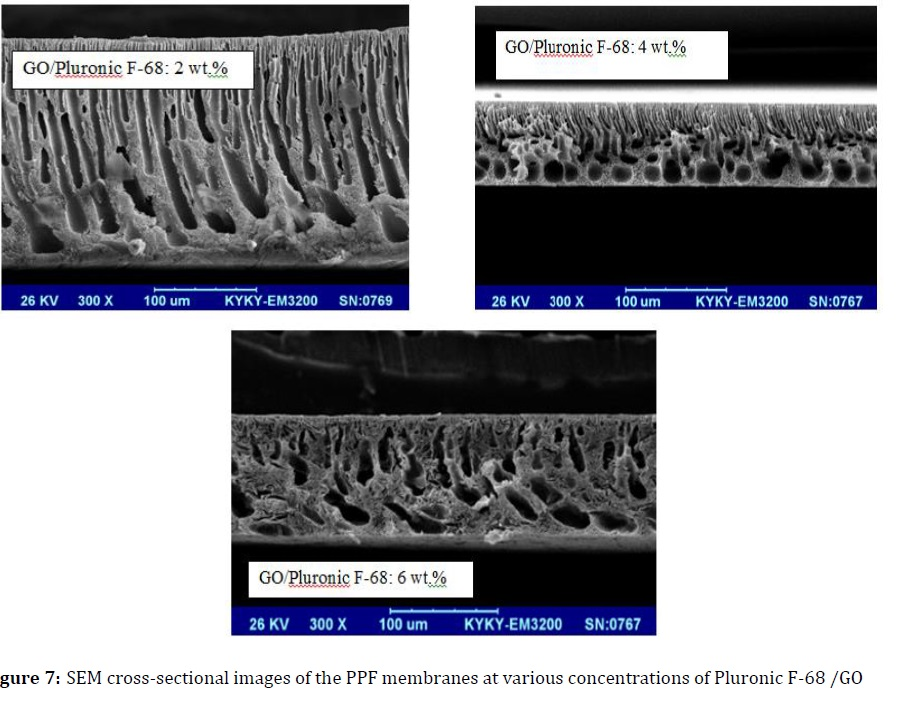Conclusion

In this research the Flory-Huggins model based on the Gibbs free energy of the casting solution was used to determine the thermodynamic stability of the membrane solution. Likewise, the effect of component interaction parameters on ternary and quaternary system was evaluated. The g123 values for ternary and quaternary system were evaluated 0.56 and 0.63, respectively. It means that the GO/PF-68 additive has the influence on the ternary interaction parameter of PPF/2P/Water in the membrane system. In fact, the hydrophilic property of GO/PF-68 makes a great tendency to non-solvent (water), so the ability of water for penetration in to nascent film increased and the exchange process of solvent/ non-solvent can be accelerated. Therefore, the thermodynamic stability was deteriorated. Results show that variations in the quaternary parameter have affected the binodal curve in the quaternary system so that in positive value of g1234, the binodal curve shift to the (polymer/additive)/ solvent axis. The GO/PF-68 additive also affects the membrane structure. At 4 wt.% of GO/PF-68, which has lower thermodynamic stability, macrovoids are regularly seen throughout the membrane. A porous structure can also be seen between these macrovoids.  At higher concentration (> 4 wt.%), due to the thermodynamic stability and higher viscosity of the casting solution, a denser structure be observed.

Funding

This research did not receive any specific grant from funding agencies in the public, commercial, or not-for-profit sectors.

Authors' contributions

All authors contributed to data analysis, drafting, and revising of the paper and agreed to be responsible for all the aspects of this work.

Conflict of Interest

We have no conflicts of interest to disclose.

Ehsan Ghorban Nezhad, Ali arastehnodeh, Susan Khosroyar, Mahmoodreza Khadangi. Thermodynamic Evaluation of Polymeric Membrane Solution: (Poly Propylene Fumarate/(Graphene Oxide/Pluronic F-68))/2-Pyrrolidone (2P)/Water Quaternary System Assessment. Chem. Methodol., 2023, 7(3) 211-222

https://doi.org/10.22034/CHEMM.2023.365490.1615

###### ##### References
. Bakeri G.H., Ismail A.F, Rahimnejad M., Matsuura T., Analysis of Polyetherimide/N-Methyl-2-Pyrrolidone/nonsolvent phase separation behavior, Journal of Polymer Research, 2014, 21:386 [Crossref], [Google scholar], [Publisher]
. Maghsoud Z., Navid Famili M.H., Madaeni S.H., Phase Diagram Calculations of Water/Tetrahydrofuran/Poly(vinyl chloride)Ternary System Based on a Compressible Regular Solution Model, Iran. Polym. J., 2010, 19:581 [Google scholar], [Publisher]
. Sadrzadeh M., Bhattacharjee S., Rational design of phase inversion membranes by tailoring thermodynamics and kinetics of casting solution using polymer additives, Journal of membrane science, 2013, 441:31 [Crossref], [Google scholar], [Publisher]
. Ayman E.G., Heba A., Sahar A., Construction of Ternary Phase Diagram and Membrane Morphology Evaluation for Polyamide/Formic acid/Water System, Australian Journal of Basic and Applied Sciences, 2012, 6:62 [Google scholar], [Publisher]
. Barzin J., Sadatnia B., Theoretical phase diagram calculation and membrane morphology evaluation for water/solvent/polyethersulfone systems, Polymer, 2007, 48:1620 [Crossref], [Google scholar], [Publisher]
. Xu L., Qiu F., Simultaneous determination of three Flory–Huggins interaction parameters in polymer/solvent/nonsolvent systems by viscosity and cloud point measurements, Polymer, 2014, 55:6795 [Crossref], [Google scholar], [Publisher]
. Emerson J.A., Toolan D.T., Howse J.R., Furst E.M., Epps III TH. Determination of solvent–polymer and polymer–polymer Flory–Huggins interaction parameters for poly (3-hexylthiophene) via solvent vapor swelling, Macromolecules, 2013, 46:6533 [Crossref], [Google scholar], [Publisher]
. Aryanti P.T.P., Ariono D., Hakim A.N., Wenten I.G., Flory–Huggins based model to determine thermodynamic property of polymeric membrane solution, Journal of Physics: Conference Series, 2018, 1090:012074 [Crossref], [Google scholar], [Publisher]
. Abdallah H., Amin A., El-Gendi A., El-Bayoumi M., Quaternary polymeric thermodynamic system and membrane formation mathematical model, ARPN Journal of Engineering and Applied Sciences, 2017, 12:2177 [Google scholar], [Publisher]
. Yin C., Dong J., Li Z., Zhang Z., Zhang Q., Ternary phase diagram and fiber morphology for nonsolvent/DMAc/polyamic acid systems, Polymer Bulletin, 2015, 72:1039 [Crossref], [Google scholar], [Publisher]
. Nivedita S., Ahamed D., Joseph S., Thermodynamic analysis of phase diagram of H2ODMF-PCL system: investigation on the influence of inorganic additives TiO2/MMT, Journal of Materials Science, 2020, 55:5431 [Crossref], [Google scholar], [Publisher]
. Sadrzadeh M., Bhattacharjee S., Rational design of phase inversion membranes by tailoring thermodynamics and kinetics of casting solution using polymer additives, Journal of membrane science, 2013, 441:31 [Crossref], [Google scholar], [Publisher]
. Ebrahimpour M., Safekordi A.A., Mousavi S.M., Heydari Nasab A., Phase separation analysis in the ternary system of poly (butylene succinate) /1,1,2,2,- tetrachloethane/non-solvent in relation to membrane formation, Bulgarian Chemical Communications, 2017, 49:389 [Google scholar], [Publisher]
. Aryanti P.T.P., Ariono D., Hakim A.N., Wenten L.G., Flory-Huggins Based Model to Determine Thermodynamic Property of Polymeric Membrane Solution, ournal of Physics: Conference Series, 2018, 1090:12074 [Crossref], [Google scholar], [Publisher]
. Mohsenpour S., Esmaeilzadeh F., Safekordi A., Tavakolmoghadam M., Rekabdar F., Hemmati M., The role of thermodynamic parameter on membrane morphology based on phase diagram, Journal of Molecular Liquids, 2016, 224:776 [Crossref], [Google scholar], [Publisher]
. Madaeni S.S., Bakhtiari L., Thermodynamic-based predictions of membrane morphology in water/dimethylsulfoxide/ polyethersulfone systems, Polymer, 2012, 53:4481 [Crossref], [Google scholar], [Publisher]
. Ma Y., Shi F., Zhao, W., Wu M., Zhang J., Ma J., Gao C., Preparation and characterization of PSf/clay nanocomposite membranes with LiCl as a pore forming additive, Desalination, 2012, 303:39 [Crossref], [Google scholar], [Publisher]
. Keshavarz L., Khansary M.A., Shirazian S., Phase diagram of ternary polymeric solutions containing nonsolvent/solvent/polymer: theoretical calculation and experimental validation, Polymer, 2015, 73:1 [Crossref], [Google scholar], [Publisher]
. Tompa H., Polymer Solutions; Butterworths: London, 1956
. Young T.H., Chuang W.Y., Thermodynamic analysis on the cononsolvency of poly (vinyl alcohol) in water–DMSO mixtures through the ternary interaction parameter, Journal of membrane science, 2002, 210:349 [Crossref], [Google scholar], [Publisher]